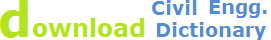absolute length correction, temperature correction, pull correction, sag correction

# Corrections for Baseline Measurement

It is necessary to apply the following corrections to the field measurements of base line order its true length:

1. Correction for absolute length
2. Correction for temperature
3. Correction of tension or pull
4. Correction for Sag
5. Correction for slope of vertical alignment

It may be noted that each section of a base line is separately corrected.

## 1. Baseline Correction for Absolute Length:

It is given by the formulaWhere CA = Correction for absolute length
L   = measured length of base
l   = Nominal length of measuring unit
C   = Correction to measuring unit

Sign of CA is the same as that of C

Nominal length: The designated length i.e 50 tape, 100 tape (30 m tape)

Absolute length: The actual length under specified conditions

## 2. Correction for Temperature:

It is given by the formulaWhere
Ct = Correction for temp= co-efficient of thermal
Tm = Mean Temperature during
To = Temp at which the measuring is standardizedSteel = 0.0000099-0.000012/cSteel = 0.0000055 – 0.0000070/ F

The sign of ‘Ct’ is plus or minus according to as ‘Tm’ is greater or less then ‘to’

## 3. Correction for Pull or TensionWhere
CP = Correction for pull
Pm = Pull applied during measurement
Po = Pull at which the measurement unit (tape) is standardized
L = length measured
A = Cross-Sectional area of measuring unit
E = Modulus of elasticity of measuring unit

E steel = 21 x 105 kg / cm2

E steel = 30 x 106 /bs/in2

The sing of this correction is always plus (T) as the effect of pull is to increase the length of the tape and consequently to decrease the measured length of the base.

## 4. Correction for Sag:

Correction for sag is the deference in length between the are and its chord i.e b/t the curved length of the laps and the distance between the supports. It’s us required only when the tape is suspended during measurement. Since the effect of sag is to make the measured length too large, it is always subtractive.

It is given by the formula.Where Cs = Correction for Sag
L1 = Distance b/t supports.
W = wt of tape per unit length
Pm = applied pullW = wt of tape b/t supports.
If there are ‘n’ equal space per tape length,
L = nL1

Sag correction per tape length isTotal sag correction to measures length

Cs=N x Cs/ + Cs//

Where N = no of whole tape length
Cs/ = Sag correction per tape length.
Cs// = Sag correction for any tape length

Normal tension:

The normal tension of a tape is a tension which will cause the effects of pull and sag to neutralize each other. It may be obtained by equating the corrections for pull and sagWhere Pn = normal tension
W = wt of tape b/w supports

Pn is determine by trial

## 5. Correction for Slope or Vertical Alignment:

This correction is required when the points of supports are not exactly at the same level
L1, L2------------ = Successive length of uniform garages
B1, b2------------ = Difference of elevation b/t the extremities of each of these grades.
Cg = corr for slope
Cg = B1C1 = AC1 – AB1 = l –DThis correction is always negative for measured length.

• If grades are given in terms in terms of vertical angleSite Map | Civil Engineering Training | Contact Us || © 2014 WebTechTix